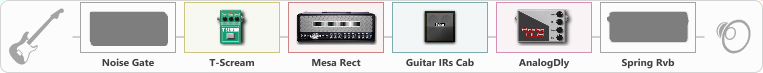Discussion in 'ToneLib-GFX presets' started by Jenselt, Feb 12, 2021.

Effects chain:Effect: "Noise Gate" (Dinámica / Filtro), active - "no"
{
"Mode" = Manual
"Depth" = 50
"Threshold" = 85
"Attack" = 0
"Hold" = 5
"Decay" = 250
}

Effect: "T-Scream" (Overdrive / Distorsión), active - "yes"
{
"Drive" = 25
"Tone" = 50
"Level" = 50
}

{
"Gain" = 50
"Bass" = 22
"Middle" = 31
"Treble" = 50
"Presence" = 72
"Master" = 64
"Level (dB)" = 0
}

Effect: "Guitar IRs Cab" (Gabinetes), active - "yes"
{
"Model" = Mesa Rectifier (4x12")
"Mic Position" = Middle
"Mic Distance" = Near
"Low Cut (Hz)" = 60
"Hi Cut (kHz)" = 20.0
"Mix" = 100
"Level (dB)" = 0
}

Effect: "AnalogDly" (Retrasar), active - "yes"
{
"Time" = 380
"Feedback" = 34
"Tone" = 65
"Mix" = 40
}

Effect: "Spring Rvb" (Reverberación), active - "no"
{
"Time" = 7.0
"PreDelay" = 0
"LoDamp" = 16
"HiDamp" = 26
"Mix" = 42
}

Note: You will need to download and install the ToneLib-GFX software to use the preset.